# Pleased to Meet You Five people meet in

• Slides: 13Pleased to Meet You • Five people meet in a room. Each one shakes hands with the other four. • How many handshakes are there? • (Hint: Draw a picture)Pleased to Meet You • There a total of 10 handshakes. • Draw five circles to represent the five people. Since each handshake is created by two people, draw lines between each of the circles to represent the handshakes. Then, count the lines to find that there a total of 10 handshakes.Geometry: Circles and Circumference Lesson 6 -9Circles • A circle is the set of all points in a plane that are the same distance from a given point, called the center.Circles The diameter (d) is the distance across a circle through the center of the circle. The circumference (C) is the distance around a circle. The radius (r) is the distance from the center to any point on the circle.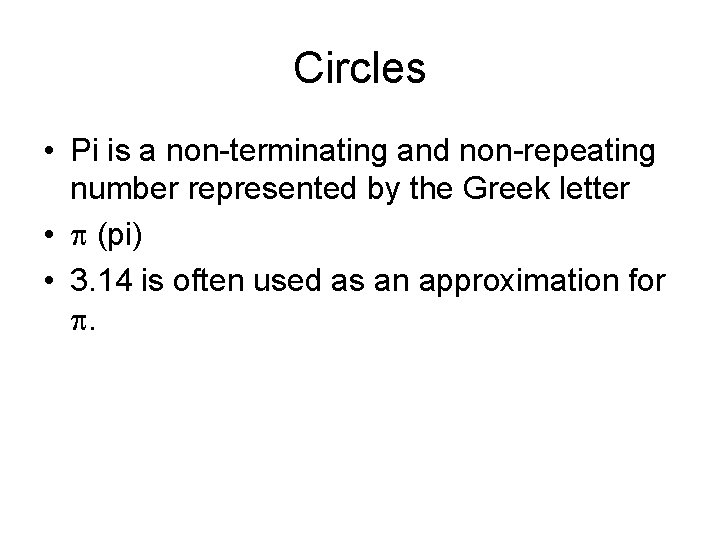Circles • Pi is a non-terminating and non-repeating number represented by the Greek letter • (pi) • 3. 14 is often used as an approximation for .Circles • • Formulas for finding Circumference C = d C = 2 r If you are given the diameter in a problem use the formula with d. If you are given a problem with the radius use the formula with r. • Both formulas find the circumference.Find Circumference • Which formula for C will you use? • C = 2 r • C = 2 (21) • C = 131. 9 inches 21 in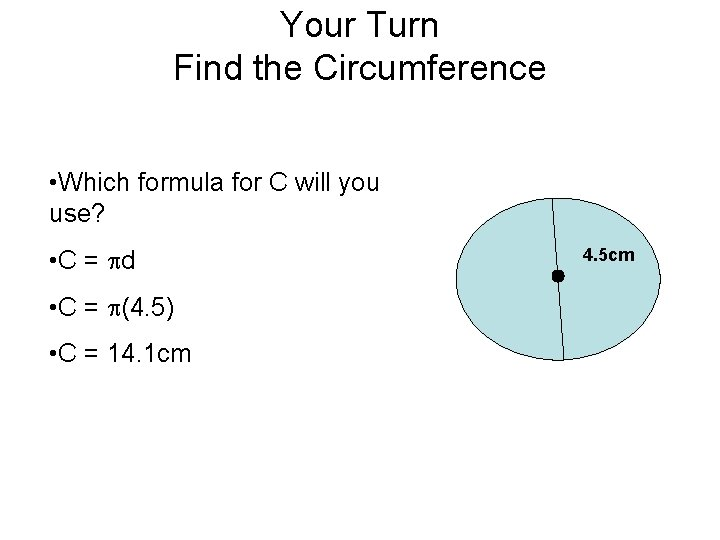Your Turn Find the Circumference • Which formula for C will you use? • C = d • C = (4. 5) • C = 14. 1 cm 4. 5 cm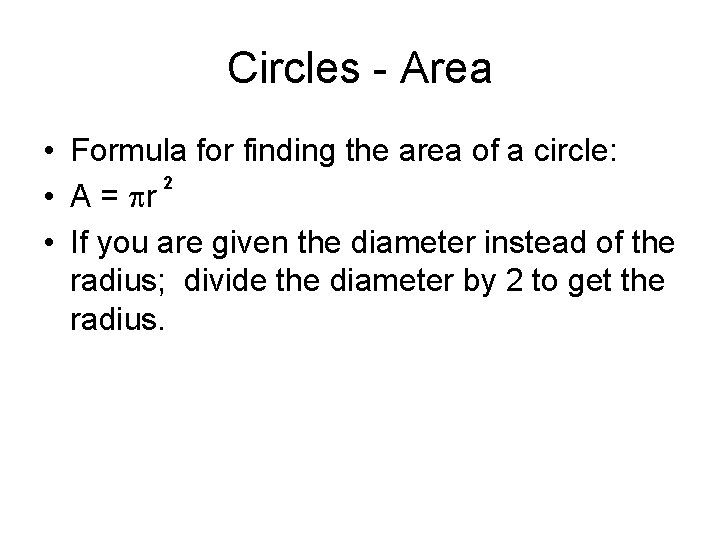Circles - Area • Formula for finding the area of a circle: 2 • A = r • If you are given the diameter instead of the radius; divide the diameter by 2 to get the radius.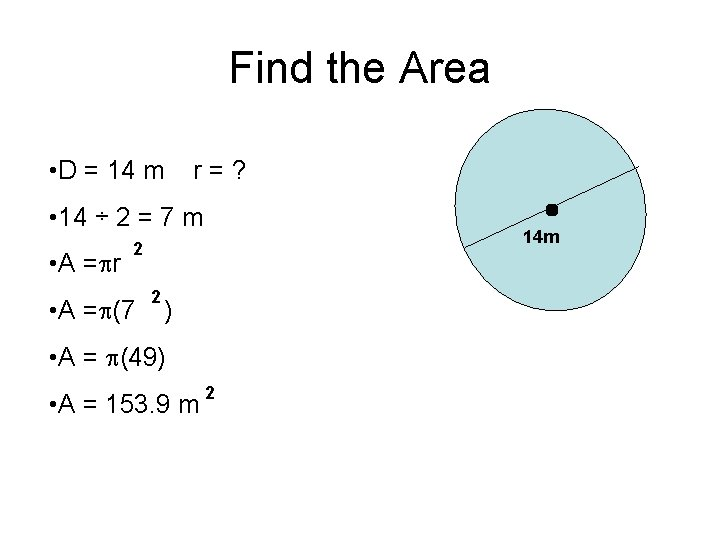Find the Area • D = 14 m r=? • 14 ÷ 2 = 7 m • A = r 14 m 2 • A = (7 2 ) • A = (49) • A = 153. 9 m 2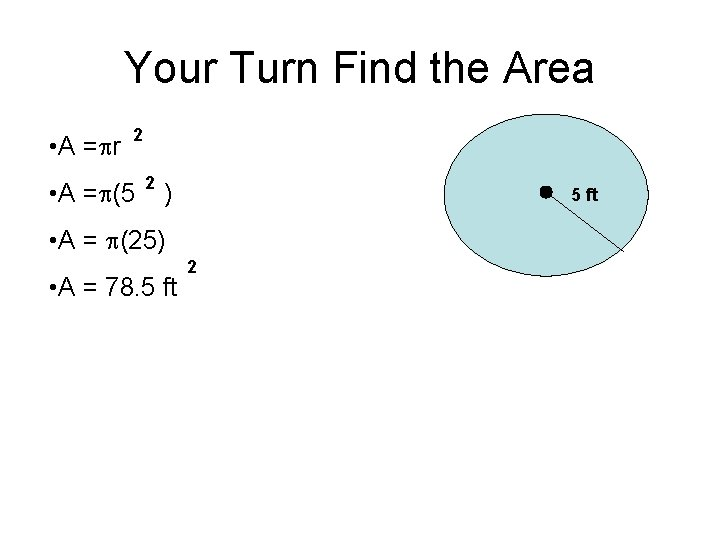Your Turn Find the Area • A = r 2 • A = (5 2 ) 5 ft • A = (25) • A = 78. 5 ft 2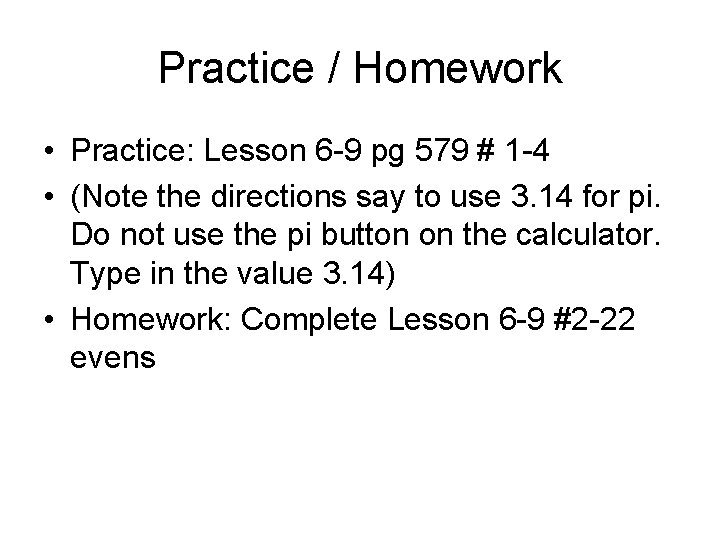Practice / Homework • Practice: Lesson 6 -9 pg 579 # 1 -4 • (Note the directions say to use 3. 14 for pi. Do not use the pi button on the calculator. Type in the value 3. 14) • Homework: Complete Lesson 6 -9 #2 -22 evens